2022: SklogWiki celebrates 15 years on-line

# Inverse Monte Carlo

Inverse Monte Carlo refers to the numerical techniques to solve the so-called inverse problem in fluids. Given the structural information (distribution functions) the inverse Monte Carlo technique tries to compute the corresponding interaction potential. More information can be found in the review by Gergely Tóth (see reference 4).

## An inverse Monte Carlo algorithm using a Wang-Landau-like algorithm

A detailed explanation of the procedure can be found in reference 1. Here an outline description for a simple fluid system is given:

#### Input information

1. The experimental radial distribution function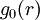$g_0(r)$ at given conditions of temperature,$T$ and density$\rho$
2. An initial guess for the effective interaction (pair) potential, i.e.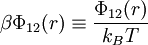$\beta \Phi_{12} (r) \equiv \frac{ \Phi_{12}(r) }{ k_B T}$

#### Procedure

The simulation procedure is divided into several stages. First, simulations are performed to modify the effective interaction at each stage,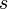$s$, in order to bias the the radial distribution function,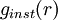$g_{inst}(r)$ towards the target$g_0(r)$ by using: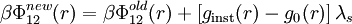$\beta \Phi_{12}^{new}(r) = \beta \Phi_{12}^{old}(r) + \left[ g_{\mathrm{inst}}(r) - g_0(r) \right] \lambda_s$,

where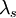$\lambda_s$ is greater than zero and depends on the stage$s$ at which one is at. The simulation for each stage proceeds until some convergence criteria (that takes into account the precision of the values of$g_0(r)$) for the global result of the radial distribution function over the stage, is achieved (See Ref. 1)) When the simulation for a particular stage have finished a new stage is initiated, with a smaller value of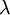$\lambda$: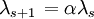$\left. \lambda_{s+1} \right.= \alpha \lambda_s$ with: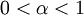$0 < \alpha < 1$

At the final stage, with a sufficiently small$\lambda$, one can obtain an effective pair potential compatible with the input radial distribution function$g_0(r)$. One knows that this effective pair potential is valid due to the uniqueness theorem of Henderson (Ref. 3).